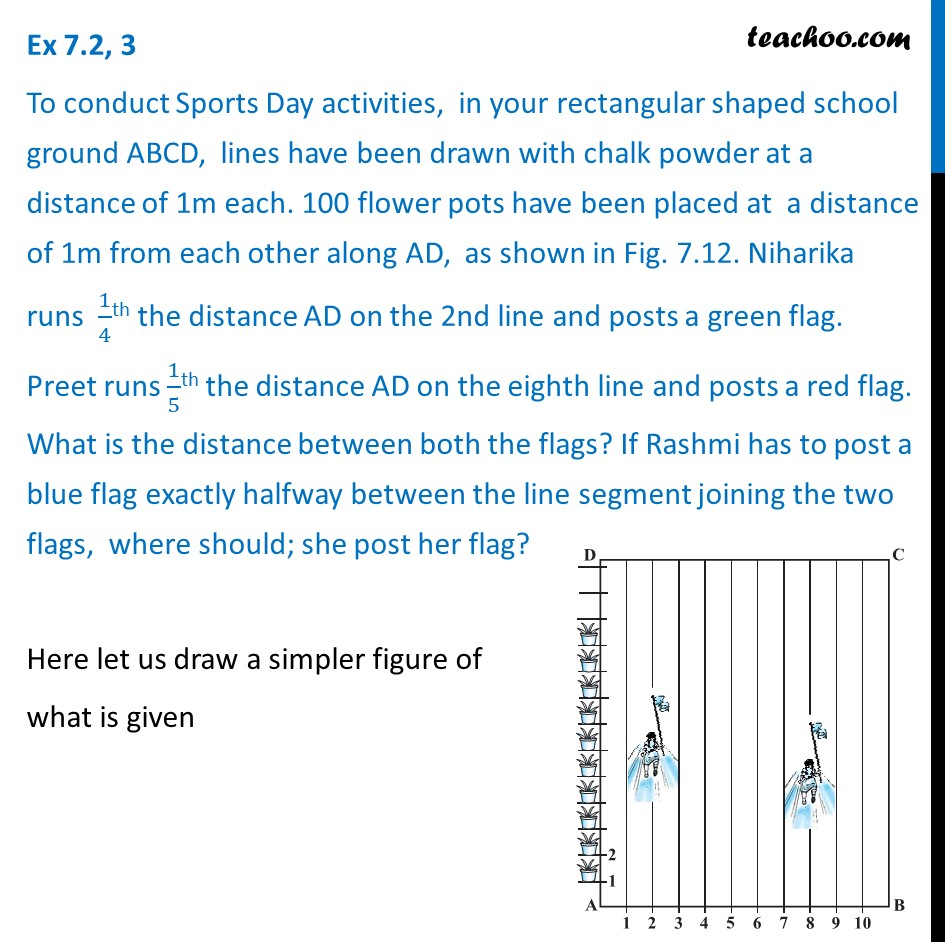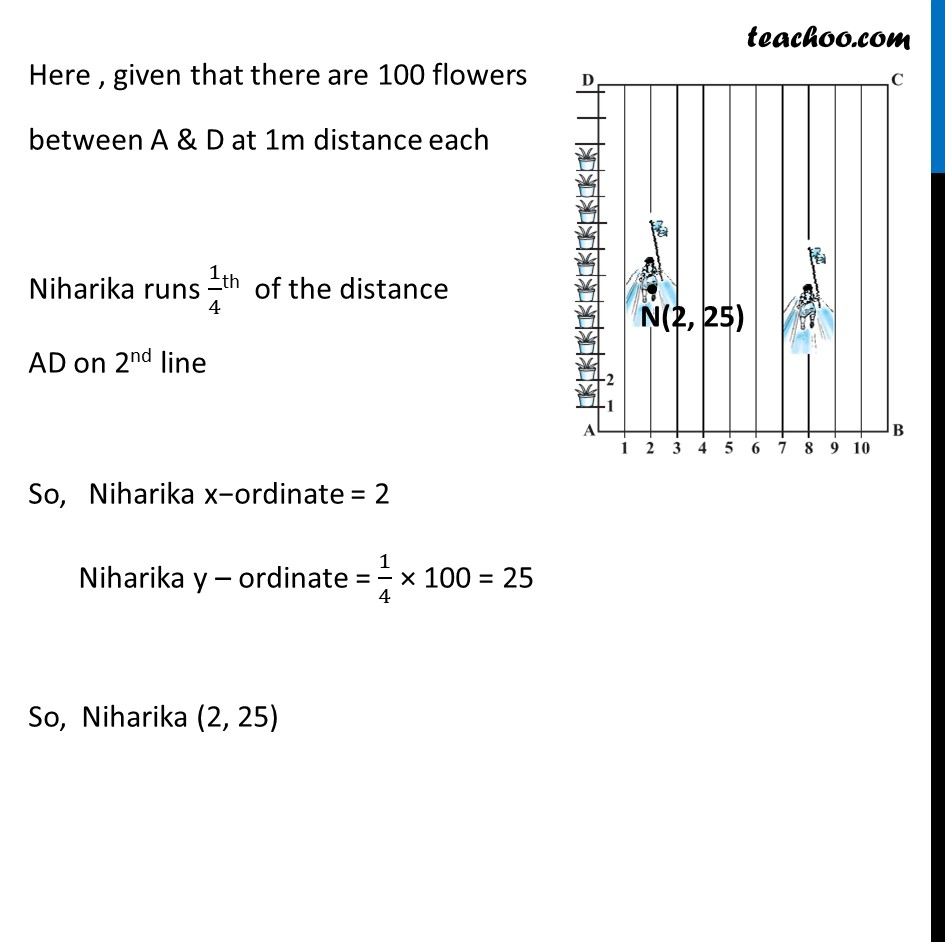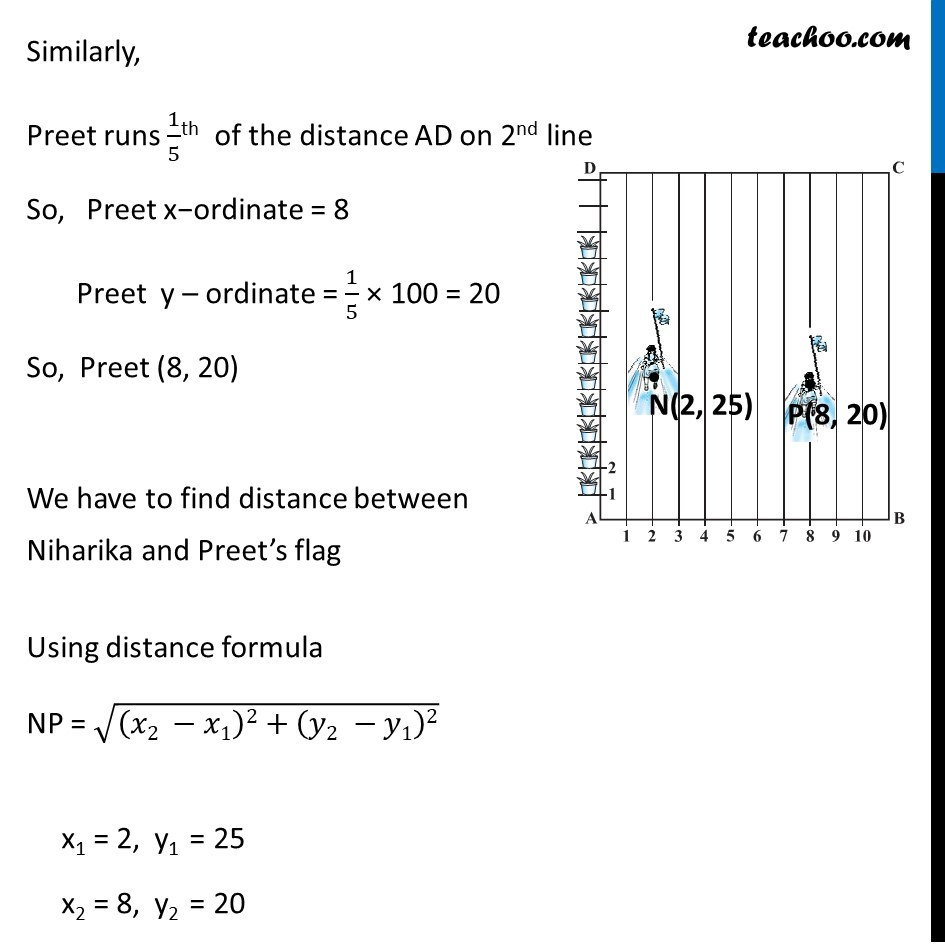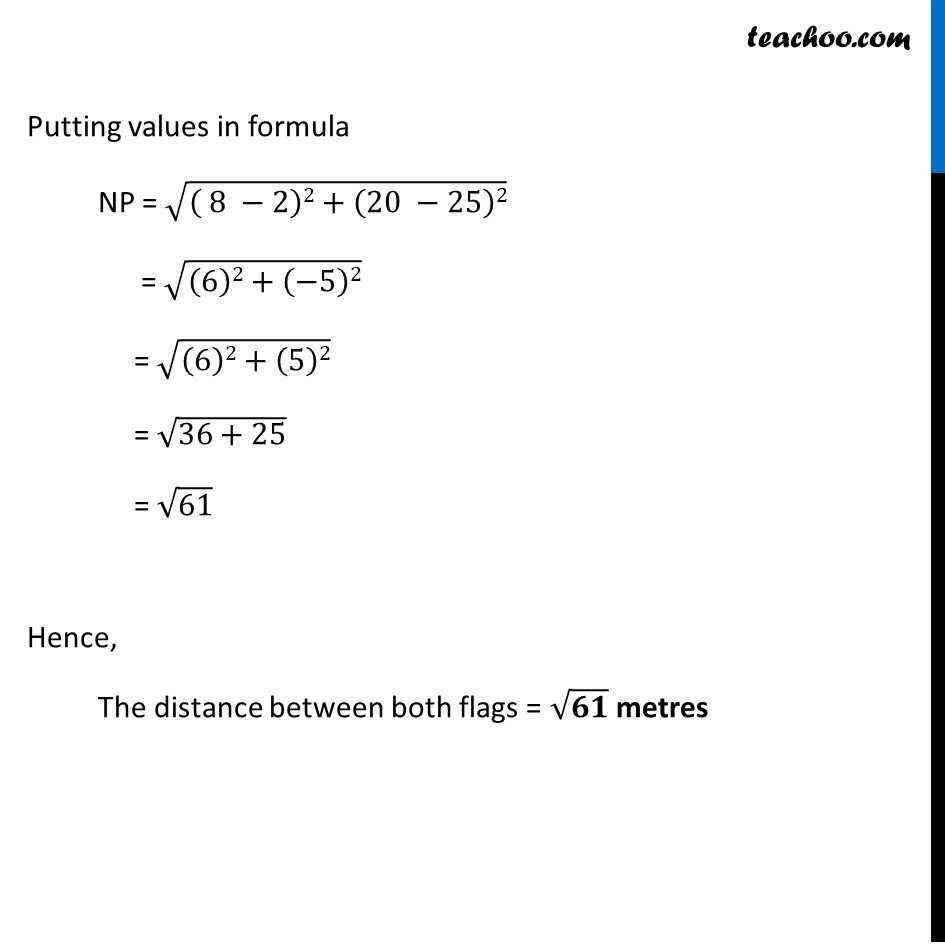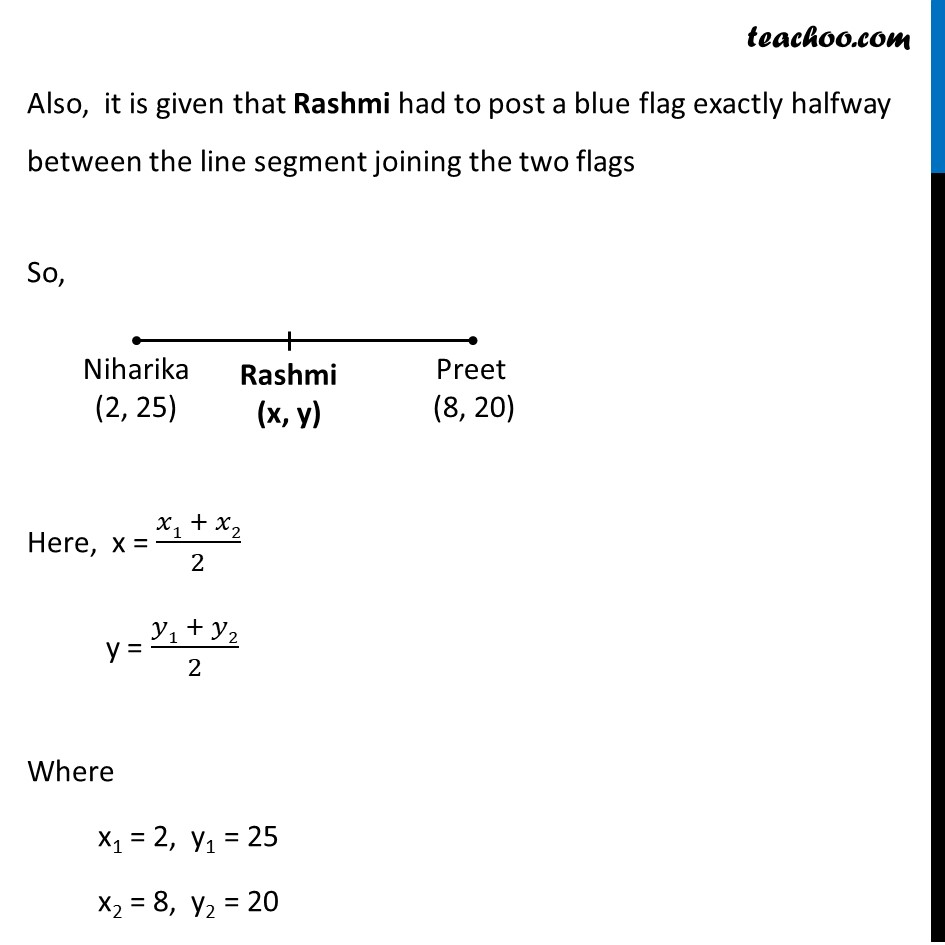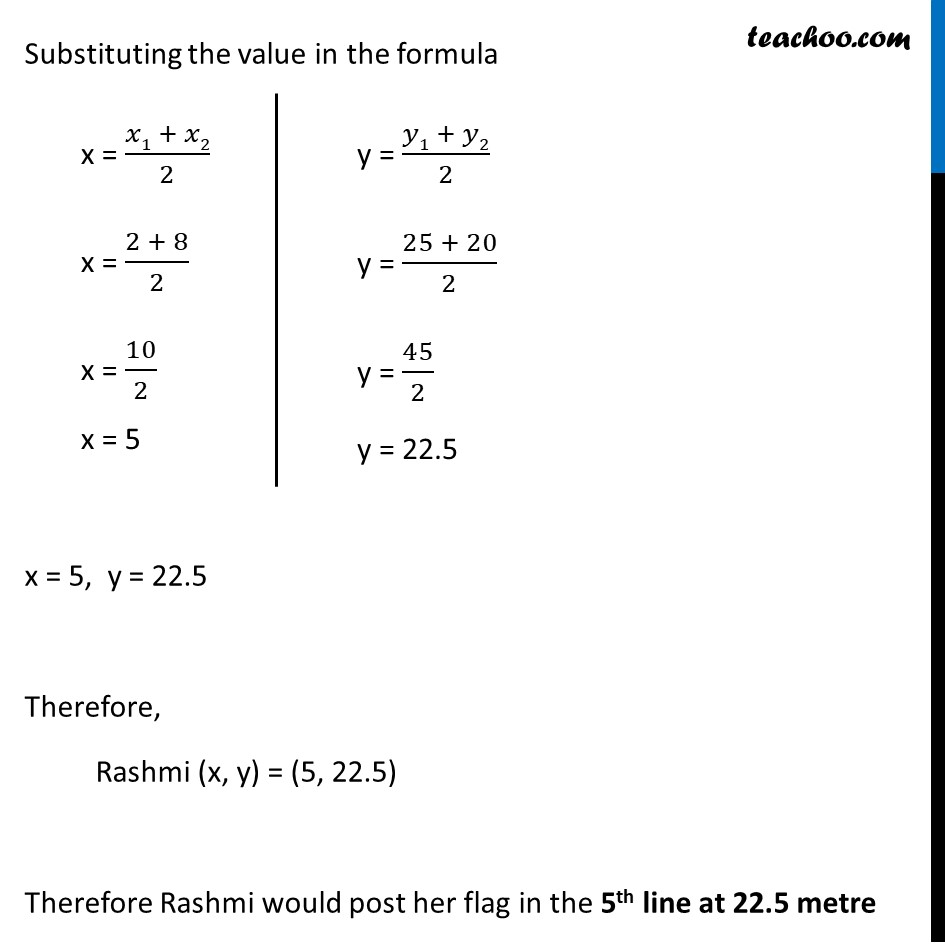1. Chapter 7 Class 10 Coordinate Geometry (Term 1)
2. Serial order wise
3. Ex 7.2

Transcript

Ex 7.2, 3 To conduct Sports Day activities, in your rectangular shaped school ground ABCD, lines have been drawn with chalk powder at a distance of 1m each. 100 flower pots have been placed at a distance of 1m from each other along AD, as shown in Fig. 7.12. Niharika runs 1/4th the distance AD on the 2nd line and posts a green flag. Preet runs 1/5th the distance AD on the eighth line and posts a red flag. What is the distance between both the flags? If Rashmi has to post a blue flag exactly halfway between the line segment joining the two flags, where should; she post her flag? Here let us draw a simpler figure of what is given Here , given that there are 100 flowers between A & D at 1m distance each Niharika runs 1/4th of the distance AD on 2nd line So, Niharika x−ordinate = 2 Niharika y – ordinate = 1/4 × 100 = 25 So, Niharika (2, 25) Similarly, Preet runs 1/5th of the distance AD on 2nd line So, Preet x−ordinate = 8 Preet y – ordinate = 1/5 × 100 = 20 So, Preet (8, 20) We have to find distance between Niharika and Preet’s flag Using distance formula NP = √((𝑥2 −𝑥1)2+(𝑦2 −𝑦1)2) x1 = 2, y1 = 25 x2 = 8, y2 = 20 Putting values in formula NP = √(( 8 −2)2+(20 −25)2) = √((6)2+(−5)2) = √((6)2+(5)2) = √(36+25) = √61 Hence, The distance between both flags = √𝟔𝟏 metres Also, it is given that Rashmi had to post a blue flag exactly halfway between the line segment joining the two flags So, Here, x = (𝑥1 + 𝑥2)/2 y = (𝑦1 + 𝑦2)/2 Where x1 = 2, y1 = 25 x2 = 8, y2 = 20 Substituting the value in the formula x = 5, y = 22.5 Therefore, Rashmi (x, y) = (5, 22.5) Therefore Rashmi would post her flag in the 5th line at 22.5 metre x = (𝑥1 + 𝑥2)/2 x = (2 + 8)/2 x = 10/2 x = 5 y = (𝑦1 + 𝑦2)/2 y = (25 + 20)/2 y = 45/2 y = 22.5